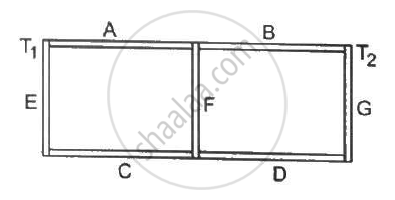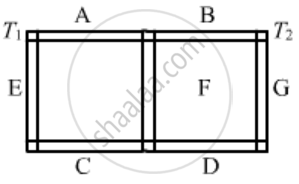Share

# Seven Rods A, B, C, D, E, F and G Are Joined as Shown in Figure (28-e9). All the Rods Have Equal Cross-sectional Area a and Length L. the Thermal Conductivities of the Rods Are Ka = Kc = K0, Kb - Physics

ConceptThermal Expansion of Solids

#### Question

Seven rods A, B, C, D, E, F and G are joined as shown in the figure. All the rods have equal cross-sectional area A and length l. The thermal conductivities of the rods are KA = KC = K0, KB = KD = 2K0, KE = 3K0, KF = 4K0 and KG = 5K0. The rod E is kept at a constant temperature T1 and the rod G is kept at a constant temperature T2 (T2 > T1). (a) Show that the rod F has a uniform temperature T = (T1 + 2T2)/3. (b) Find the rate of heat flowing from the source which maintains the temperature T2.#### SolutionGiven:

KA = KC = K0

KB = KD = 2K0

KE = 3K0, KF = 4K0

K9= 5K0

Here, K denotes the thermal conductivity of the respective rods.

In steady state, temperature at the ends of rod F will be same.

Rate of flow of heat through rod A + rod C = Rate of flow of heat through rod B + rod D

(K_A·A·(T-T_1))/ ( l ) + (K_c·A(T-T_1))/(l) +  (K_B·A( T_2 - T ))/l

2k0 ( T - T) = 2 × 2 K0 ( T2 - T)

Temp of rod F = T =  (T_1 + 2T_2)/3

(b) To find the rate of flow of heat from the source (rod G), which maintains a temperature T2 is given by

Rate of flow of heat, q = (DeltaT)/("Thermal resistance")

First, we will find the effective thermal resistance of the circuit.

From the diagram, we can see that it forms a balanced Wheatstone bridge.

Also, as the ends of rod F are maintained at the same temperature, no heat current flows through rod F.

Hence, for simplification, we can remove this branch.

From the diagram, we find that Rand RB are connected in series.

∴ RAB = RA + RB

RC and Rare also connected in series.

∴ RCD = RC + RD

Then, RAB and RCD are in parallel connection.

R_A  = l/(K_0A)

R_B = l/(2K_0A)

R_C = l /( K_0A)

R_D = l/(2K_0A)

R_{AB} = (3l)/(2k_0A)

R_{CD} = (3l)/(2K_0A)

R_"eff" = ((3l)/(2K_0A)xx(3l)/(2K_0A))/ (((3l)) /( 2K_0A) + (3l)/(2K_0A))

=(3l)/(4K_0A)

⇒ q = (DeltaT)/("R_eff")

= (T_1 - T_2)/((3l)/ (4K_0A))

⇒ (4K_0A(T_1 - T_2))/(3L)

Is there an error in this question or solution?

#### APPEARS IN

Solution Seven Rods A, B, C, D, E, F and G Are Joined as Shown in Figure (28-e9). All the Rods Have Equal Cross-sectional Area a and Length L. the Thermal Conductivities of the Rods Are Ka = Kc = K0, Kb Concept: Thermal Expansion of Solids.
S